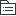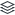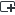# 高中数学必修一函数和基本初等函数考前要点必看

420
|
135

## 相关模板推荐

•## 函数 —— 作品大纲

•函数三要素
• 定义域
• 对应关系
• 换元法(注意把换元后t的取值范围写出来)
• 待定系数法（已知解析式类型，一次函数，二次函数等）
• 解方程组法（f(x)和f(-x)、f(x)和f(1/x)成对出现）
• 值域
•单调性（最值）
• 作差法证明，同号递增，异号递减
• 复合函数单调性（f(g(x))同增异减）
• 抽象函数单调性（和型、积型如何转化）
• 单调性求解最值得出函数值域
• 分段函数单调性（各部分解析式单调性，注意端点衔接处！！！）
•奇偶性
• 关于原点对称为奇函数，奇函数若在x=0处有定义，则f(0)=0
• 关于y轴对称为偶函数
• 函数奇偶性证明（f(-x)=-f(x)还可变换为f(x)+f(-x)=0或f(x)/f(-x)=-1，常见于指数型函数或对数型函数）
• 抽象函数的奇偶性（令y=-x，然后赋值求解剩余f(a)）
•图象及其变换
• 左加右减
• 上加下减
•基本初等函数
• 指数函数
• (指数函数常考点：值域（0，+∞）)
• 对数函数
• y=logaX（a>0，且a≠1）(对数函数常考点：定义域（0，+∞）)
• 必考：对数式运算（基本运算公式、换底公式应用）
• 幂函数
• 熟记常见基本幂函数图象！
• 注意与指数函数表达式区别
•集合
• 集合元素的特性
• 确定性
• 互异性
• 无序性
• 元素与集合之间的关系
• 属于
• 不属于
• 集合间的关系
• ⫋（能写真包含于不写包含于）
• 空集Ø
• 空集是任何集合的子集！！！
• 集合之间的运算
• 交集A∩B
• 并集A∪B
• Venn图、数轴解答（注意端点！！！）
• 补集∁uA
• 集合的含义
• 点集{（x,y）}
• 数集(表示定义域{x|f(x)}、表示值域{y|f(x)}傻傻分不清楚)